# EEG speech envelope TRF

Analyze continuous speech data from the mTRF dataset 1: use the boosting algorithm for estimating temporal response functions (TRFs) to the acoustic envelope.

```# Author: Christian Brodbeck <christianbrodbeck@nyu.edu>
# sphinx_gallery_thumbnail_number = 4
import os

import mne
from eelbrain import *

# Load the mTRF speech dataset and convert data to NDVars
root = mne.datasets.mtrf.data_path()
speech_path = os.path.join(root, 'speech_data.mat')

# Time axis
tstep = 1. / mdata['Fs'][0, 0]
n_times = mdata['envelope'].shape
time = UTS(0, tstep, n_times)
# Load the EEG sensor coordinates (drop fiducials coordinates, which are stored
# after sensor 128)
sensor = Sensor.from_montage('biosemi128')[:128]
# Frequency dimension for the spectrogram
band = Scalar('frequency', range(16))
# Create variables
envelope = NDVar(mdata['envelope'][:, 0], (time,), name='envelope')
eeg = NDVar(mdata['EEG'], (time, sensor), name='EEG', info={'unit': 'µV'})
spectrogram = NDVar(mdata['spectrogram'], (time, band), name='spectrogram')
eeg = eeg[sensor.index(exclude='A13')]
```

## Data

Plot the spectrogram of the speech stimulus:

```plot.Array(spectrogram, xlim=5, w=6, h=2)
```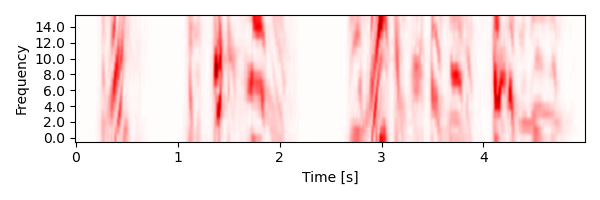```<Array: spectrogram>
```

Plot the envelope used as stimulus representation for deconvolution:

```plot.UTS(envelope, xlim=5, w=6, h=2)
```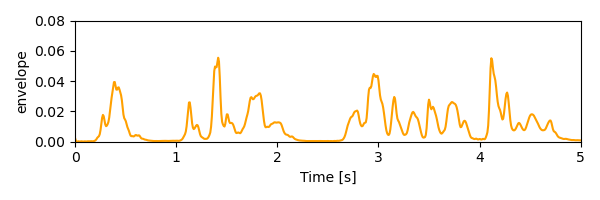```<UTS: envelope>
```

Plot the corresponding EEG data:

```p = plot.TopoButterfly(eeg, xlim=5, w=7, h=2)
p.set_time(1.200)
```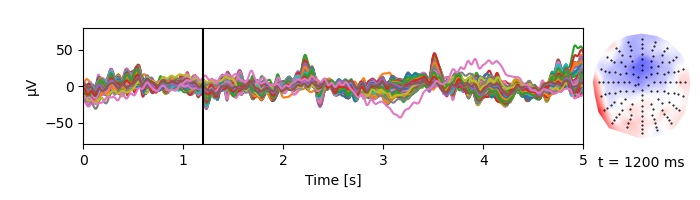## Deconvolution

TRF for the envelope using boosting:

• TRF from -100 to 400 ms

• Basis of 100 ms Hamming windows

• Use 4 partitionings of the data for cross-validation based early stopping

```res = boosting(eeg, envelope, -0.100, 0.400, basis=0.100, partitions=4)
p = plot.TopoButterfly(res.h_scaled, w=6, h=2)
p.set_time(.180)
```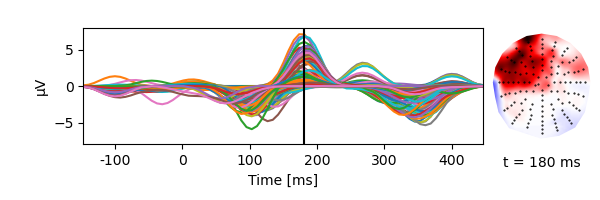## Multiple predictors

Multiple predictors additively explain the signal:

```# Derive acoustic onsets from the envelope
onset = envelope.diff('time', name='onset').clip(0)
onset *= envelope.max() / onset.max()
plot.UTS([[envelope, onset]], xlim=5, w=6, h=2)
```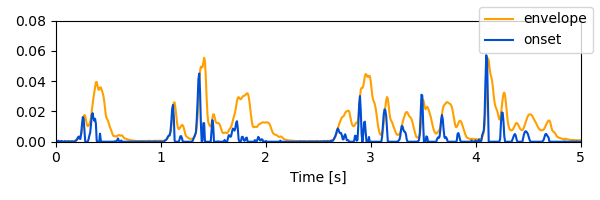```<UTS: envelope, onset>
```
```res_onset = boosting(eeg, [onset, envelope], -0.100, 0.400, basis=0.100, partitions=4)
p = plot.TopoButterfly(res_onset.h_scaled, w=6, h=3)
p.set_time(.150)
```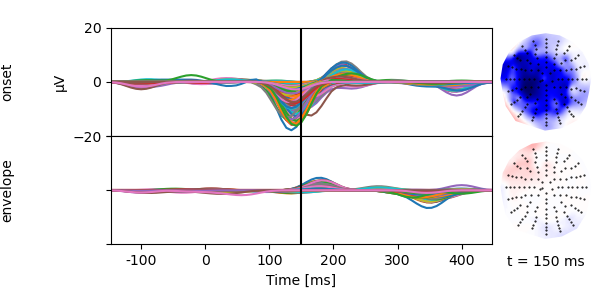## Compare models

Compare model quality through the correlation between measured and predicted responses:

```plot.Topomap([res.r, res_onset.r], w=4, h=2, ncol=2, axtitle=['envelope', 'envelope + onset'])
```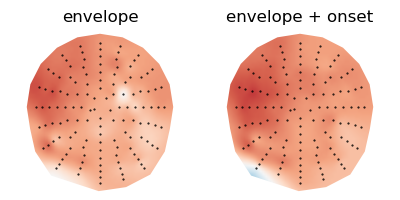```<Topomap: Correlation>
```